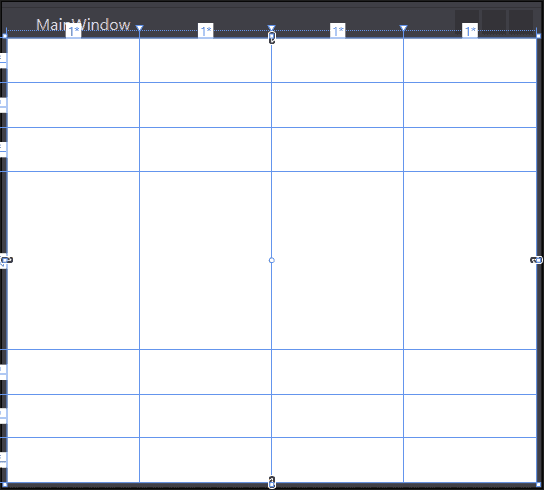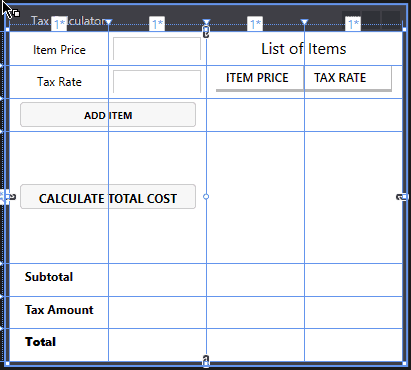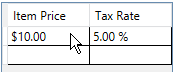# How to Create a Simple Tax Calculator WPF Application in C#

In my last tutorial, I showed you how to create a simple tax calculator console application in C#. As promised, in this tutorial I will show you how to make that same tax calculator with a Graphical User Interface (GUI) and with a few feature enhancements. By the end of this tutorial, you will be able to run your tax calculator and calculate the grand total of a list of items with different tax rates for each item.

## Requirements

Difficulty: Easy

Concepts Used:

We want to create a GUI application that can calculate the sales tax and grand total of a list of items that are input from the user. Each item in this list of items will have the ability to be taxed at a different tax rate. We then display these calculations on the screen to the user.

## Let’s Get Started

To get started, we need to create an empty C# WPF application in Visual Studio. For more information on how to do this click here.

### The User Interface (UI)

Now that we have our new blank WPF application, we are ready to get started.

#### The Grid Layout

First, in the MainWindow.xaml file set the Height equal to 360 and the Width equal to 400. Now inside the grid element we are going to add rows and columns. Take a look at the code below to see how this is done.

```<Grid.ColumnDefinitions>
<ColumnDefinition Width="*"/>
<ColumnDefinition Width="*"/>
<ColumnDefinition Width="*"/>
<ColumnDefinition Width="*"/>
</Grid.ColumnDefinitions>

<Grid.RowDefinitions>
<RowDefinition Height="*"/>
<RowDefinition Height="*"/>
<RowDefinition Height="*"/>
<RowDefinition Height="4*"/>
<RowDefinition Height="*"/>
<RowDefinition Height="*"/>
<RowDefinition Height="*"/>
</Grid.RowDefinitions>```

Above you will see that we are creating 4 columns all the same width. We know this because all of the columns have a Width property is set to * which means split the available space evenly among the elements.

For the Rows, we have seven rows with the 4th row 4 times bigger than the rest of the rows. We know this because the 4th row has its width property set to 4*. This is interpreted to 4/7th of the available space.

Once you have the grid layout in the designer your MainWindow should have guidelines that look like this:#### The Inputs

Now that we have the grid layout the way we want, it’s time to start adding the elements to the grid. The first element we are going to add is a label with a name of “ItemPriceLabel” and centered. We want the label to have a content of “Item Price”. See below for how to do this.

`<Label x:Name="ItemPriceLabel" Content="Item Price" VerticalAlignment="center" HorizontalAlignment="Center" />`

After the label we want to add a text box in the second column with a name of “ItemPriceInput” and a 5px margin all the way around it.

`<TextBox x:Name="ItemPriceInput" Grid.Column="1" Margin="5 5 5 5" />`

Note in the above code we have a property Grid.Column=“1”. This means we want to put the element in the second column in the window since the columns and rows are zero-based or start counting from zero. The default is 0 which is why we didn’t hear to put this on the label before.

Let’s repeat the above step for the Tax Rate label and input.

```<Label Content="Tax Rate" Grid.Row="1"  VerticalAlignment="Center" HorizontalAlignment="Center" />
<TextBox x:Name="TaxRateInput"  Grid.Column="1" Grid.Row="1" Margin="5 5 5 5" />```

Notice in the above code we added a Grid.Row=“1” which puts the elements in the second row from the top.

#### The Buttons

Now were are going to add a button for adding an Item. We want the button to be 20px tall and have a 10px margin on the sides while reaching across the first and second columns. Check out the code below.

`<Button x:Name="AddItemButton" Content="Add Item" Height="20" Grid.Row="2" Grid.ColumnSpan="2" Margin="10 0 10 0" />`

Notice the Grid.ColumnSpan=“2” which tells the element to reach or span across 2 columns starting in the column it is placed.

Now another button for calculating the total price. We want this button to have a font size of 12px and bold.

`<Button x:Name="CalculateButton" Content="Calculate Total Cost" Height="20" FontSize="12" Grid.ColumnSpan="2" Grid.Column="0" Grid.Row="3" Margin="10 0 10 0" FontWeight="Bold" />`

#### The Calculations

Now let’s add three more labels just like before in the first column and last three rows. Also, we are going to add three text boxes with no content in the second column beside the labels.

```<Label Content="Subtotal" Grid.Row="4" Margin="10 0 0 0" FontWeight="Bold" />
<TextBlock x:Name="Subtotal" Grid.Row="4" Grid.Column="1" />

<Label Content="Tax Amount" Grid.Row="5" Margin="10 0 0 0" FontWeight="Bold" />
<TextBlock x:Name="TaxAmount" Grid.Row="5" Grid.Column="1"/>

<Label Content="Total" Grid.Row="6" Margin="10 0 0 0" FontWeight="ExtraBold" />
<TextBlock x:Name="TotalPrice" Grid.Row="6" Grid.Column="1" FontWeight="Bold" />```

#### Displaying the Data

On the other side at the top of the MainWindow, we want to add a label that is centered across the 3rd and 4th columns that say “List of Items” in a font size of 16px. Do you remember how to do this? Before taking a look at the code below give it a try for yourself the check what you have with what I have below.

`<Label Content="List of Items" Grid.Column="2" Grid.ColumnSpan="2" FontSize="16" VerticalAlignment="Center" HorizontalAlignment="Center" />`

Did you have it right? If so, congrats. If not, that’s ok you will get the hang of this.

As we add items to the list, we will need a way to display that list. There is more than one way to do this, but in this tutorial, we will be using a data grid for displaying the prices and tax rates that are in the list. See the code below to see how to do this

```<DataGrid x:Name="ItemsDataGrid" Grid.Row="1" Grid.Column="2" Grid.ColumnSpan="2" Grid.RowSpan="5" Margin="10 0 10 0" AutoGenerateColumns="false" HorizontalAlignment="Center">
<DataGrid.Columns>
</DataGrid.Columns>
</DataGrid>```

In the above code, you will see that we have created a DataGrid element with two DataGridColumns. We use the Header property to give the data columns a header and the Width property set to “*” to split the two columns evenly in the available space. There are a few more things we will need to do to this DataGrid to get it working properly, but we will come back to this later when things make more sense.

At this point, the UI should look like the Image shown below.### The Code Behind

It’s time to move on to the code behind for the UI which is located in the file “MainWindow.xaml.cs”. We can get there by going to the solution explorer and clicking the dropdown beside MainWindow.xaml. Here you will find the file labeled “MainWindow.xaml.cs”.

The first thing we want to do is create a method that gets run when the “Add Item” button is clicked. The easiest way to do this is to go to the MainWindow.xaml file and in the designer window double-click the “Add Item” button. This will add a Property Click=“AddItemButton_Click” and will also create this method in the MainWindow.xaml.cs file.

Inside the newly created “AddItemButton_Click” method we are going to add the following code.

```try
{
decimal itemPrice = Decimal.Parse(ItemPriceInput.Text);
decimal taxRate = Decimal.Parse(TaxRateInput.Text)/100;
}
catch (Exception)
{
MessageBox.Show("Please enter a valid amount in item price and tax rate");
}```

Above you will see we create a try-catch block. In the try block, we try to parse the user input and convert it to a decimal. If the user enters something other than numbers the try will fail and the catch will execute causing a message box to pop up and tell the user to “Please enter a valid amount in item price and tax rate”. If the conversion succeeds, the try block will call the “AddNewItem” method (which has not been created yet) passing the item price and tax rate.

Before creating the AddNewItem method, we need to do a couple of things. We create a class “Item” which has two properties, ItemPrice and TaxRate, which have get and set methods as shown below.

```public class Item
{
public decimal ItemPrice { get; set; }
public decimal TaxRate { get; set; }
}```

Then we create a list to hold our Items we create in the application.

`private List<Item> Items = new List<Item>();`

Now it’s time to add the AddNewItem method. Check out the code below.

```private void AddNewItem(decimal itemPrice, decimal taxRate)
{
Item item = new Item()
{
ItemPrice = itemPrice,
TaxRate = taxRate
};
}```

In the above code, we are creating a new Item and assigning the properties the corresponding values which are passed in as parameters. We then call the Add method of the List “Items” to add the Item to the list.

#### Binding the List

So now it’s time to link or bind the List “Items” to the DataGrid in the UI. To do this first, we need to make our List “Items” be an ObservableCollection. An ObservableCollection will trigger an event any time the list changes which will update the UI. To do this make the List look like the code below.

`private ObservableCollection<Item> Items = new ObservableCollection<Item>();`

Find the MainWindow method and set the ItemsDataGrid.ItemSource to be the Items list we created like this ItemsDataGrid.ItemsSource = Items; .

Back in the “MainWindow.xaml” file, we will add a property “Binding” to bind the list and the columns. Take a look at the code below to see how this should look.

```<DataGridTextColumn Header="Item Price" Width="*" Binding="{Binding ItemPrice, StringFormat=C}" />
<DataGridTextColumn Header="Tax Rate" Width="*" Binding="{Binding TaxRate, StringFormat=P}"/>```

Notice the StringFormat=C and P. This is to format the text as Currency and Percentage when displayed in the Columns.

At this point, if you run the application you will be able to add items and their tax rates to the list on the right side as shown in the image below.#### Calculate Tax Method

For the final piece, we are going to implement the calculate total price button. Like before we can do this by double-clicking the Calculate Total Cost button. In the generated method we will add three local variables, subtotal, total, and taxAmount.

Then we will add a foreach loop to iterate over all of the items in the Items collection. In the foreach loop, we will add the item’s price to the subtotal variable. We will add the item’s tax amount to the taxAmount variable. Last we will add the item’s price and its tax amount to the total variable.

After the foreach loop, we will set the UI text block elements to be the value of these three elements as currency formatted strings. Take a look at the code below to see how the “CalculateButton_Click” method should look.

```private void CalculateButton_Click(object sender, RoutedEventArgs e)
{
decimal subtotal = 0;
decimal total = 0;
decimal taxAmount = 0;

foreach (var item in Items)
{
subtotal += item.ItemPrice;
taxAmount += item.ItemPrice * item.TaxRate;
total += (item.TaxRate * item.ItemPrice) + item.ItemPrice;
}

Subtotal.Text = String.Format("{0:C}", subtotal);
TaxAmount.Text = String.Format("{0:C}", taxAmount);
TotalPrice.Text = String.Format("{0:C}", total);
}```

## See it in Action

Now if we run the program we will see that everything works fine. There is, however, one annoyance. When the user adds an item the Item Price and Tax Rate inputs do not clear. We can fix this by calling the Clear(); method on each of these inputs after the try-catch block in the AddItemButton_Click method.

```ItemPriceInput.Clear();
TaxRateInput.Clear();```

Below is a final screenshot of what our simple tax calculator should look like when it is used.You did it! You now have a working tax calculator build using WPF with C#. You also have a basic understanding of building simple calculation applications with a full GUI.

Remember I am documenting my journey of learning C# by writing these tutorials, so if you see a mistake or know a better way of doing it then, please let me know in the comments below. Also, if you have any questions be sure to ask in the comments section below and I will do my best to answer.